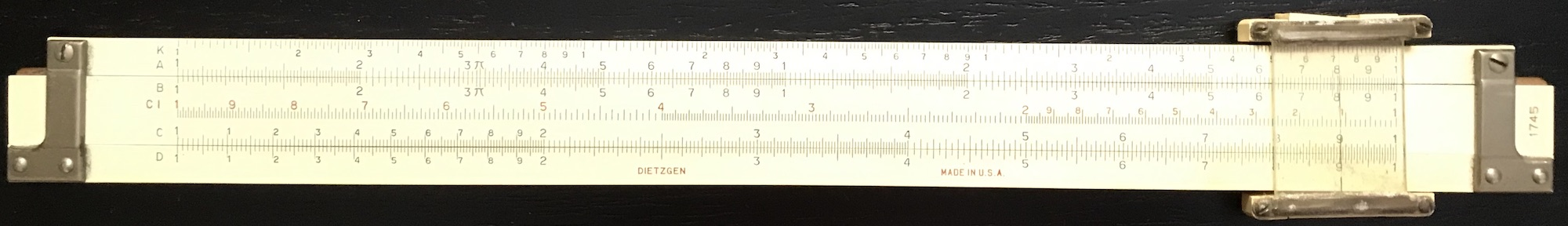## 3.4 Cubes and Cube Roots – the K Scale

The Mannheim slide rule became very popular after its standardization in the late 1800’s and soon rule makers began adding other scales to ease various computations. One that is often found on later rules is the K scale, used for cubes and cube roots. The K scale is similar to the A scale in that it is just a logarithmic scale that repeats three times over the length of the standard C scale, rather than just twice. By the same type of argument as made above for the A scale, the K scale shows the cube of a number found on the D scale; conversely, lining up the cursor on a number on the K scale, one can find that number’s cube root directly on the D scale. Inspection of the image below, which shows a Deitzgen slide rule with K, A/B, and C/D scales, can be used to illustrate the point.A Dietzgen slide rule with K, A/B, and C/D scales (plus an inverted scale, CI).

For practice, let’s repeat our calculation of the volume of a sphere of radius 4.58 inches, as was performed during our review of logarithms, but here using a slide rule. Using the C/D scales, we can compute the value of $$4\pi/3$$, which should be about 4.189. Some slide rules have this value engraved as a Gauge Mark on the K scale. The user next moves the 1 on the C scale to line up with this value on the K scale using the cursor. Then the cursor is moved along the C scale to the value of the radius of the sphere, which is 4.58 in our case. The volume can be read directly on the K scale, which should be roughly 400 cubic inches. Using the K scale the result may only be accurate to two digits for our example.